# Use Cases

## Use Case: Debugging a Formula

Spreadsheet formulas can be hard to get right, even when copied from published definitions, like this one for the familiar bell-shaped curve.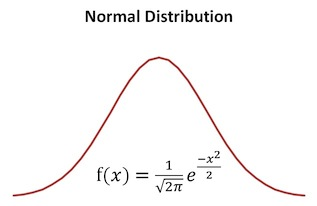Mathematical definition of bell curve

This spreadsheet shown below tries to plot the bell-shaped curve, but gets it seriously wrong.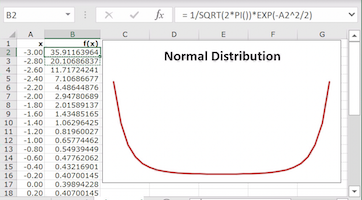Problematic transcription into Excel

Formula Forge helps users find what causes problems like this. To use it, select cell `B2` and click the `Get Formula` button on Excel's `Formulas` ribbon to see a `Formula Forge` pane with three views of the formula in cell `B2`. (The width of the pane shown here was increased by dragging its edge to the left.)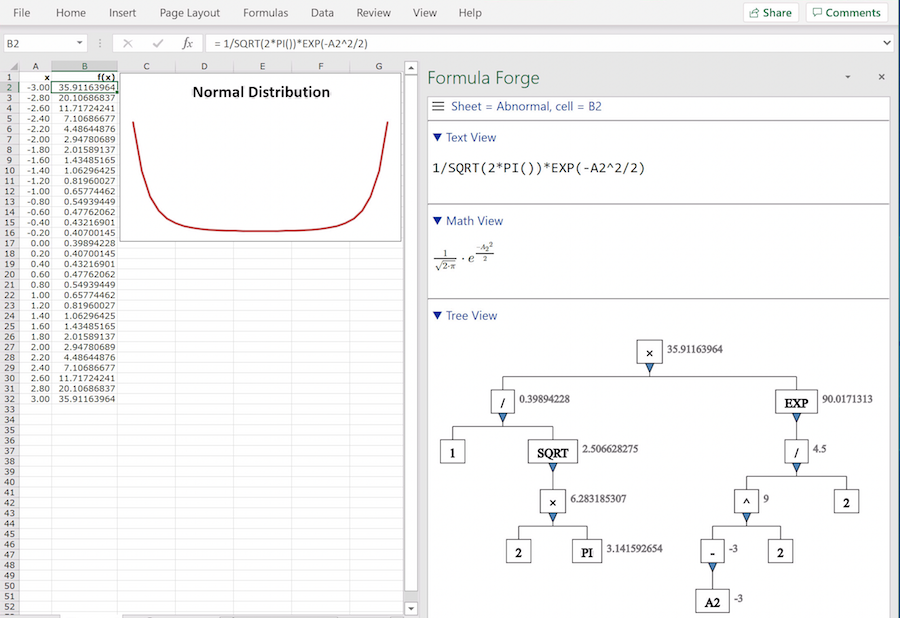View of problematic formula

• The text view shows the formula's text, as it appears in Excel's formula bar. Nothing looks wrong in this view.
• The math view typesets the formula, making it much easier to read. Nothing looks wrong in this view either.
• The tree view shows how Excel decomposes the formula into smaller expressions, along with the values of those expressions. The top value looks decidedly wrong.

We trace the origin of this incorrect value by looking at other values lower in the tree view. The problem becomes clear when we examine the box containing the unary minus sign in `-A2^2`: Excel has computed `-A2` and then squared the result. We expected it to square `A2` first, as is customary in ordinary mathematical usage and most programming languages.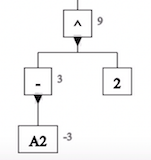Excel decomposition of -A2^2

We fix the problem by using Excel's formula bar to enclose `A2^2` in parentheses. To check that this solves the problem, we click the `Get Formula` button again to display the new version of the formula in cell `B2`. Now the top value looks right in the tree view.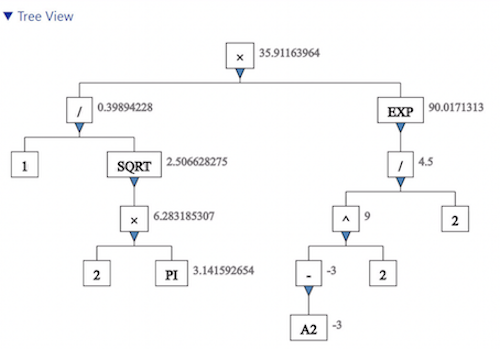Tree view of corrected formula

We finish the repair by copying the corrected formula down from cell `B2` through cell `B32` and see that it does indeed produce the expected bell-shaped curve.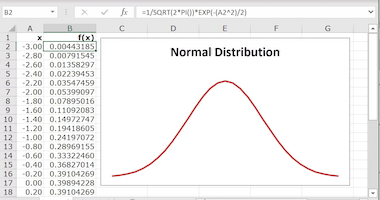Corrected transcription into Excel